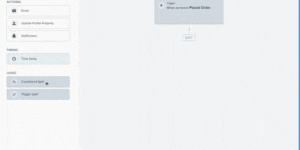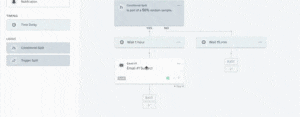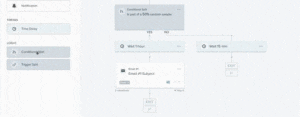# How to Split Test Flows (aka Email Sequences) in KlaviyoWritten by Andriy BoychukIn this article, we share a step-by-step tutorial on how you can split test the path of your email automations in Klaviyo.

The success of your email sequences often depends on when you send them. That’s why in this tutorial, we especially focus on how to split test time delays.

We know setting up your Klaviyo account can be tricky. That’s why we created a Klaviyo Setup Checklist – so you don’t miss any important steps in the setup process.

So , first of all, let’s go to Klaviyo. So let’s create a flow. Start from scratch. Test. And why it’s important, because let’s say if you want to create delay, so let’s do metrics. Let’s do place order, for example. Done. So now let’s say you want to split test when to send that email, like 15 minutes later or one hour later, and see what kind of results you have.I have another video called “How to A/B Test Your Emails“. And there’s a whole strategy how to do the split test the proper way. So anyway, so if you have a trigger or somebody placed an order, and let’s assume we want to split the time delays. So conditional split, trigger split. So we do conditional split here, and what we do, we do random sample, and if you want to do split test 50/50 you do, you do 50/50, but if you want to do other split tests, of course you need to strategize, and I’ll explain what do I mean.So let’s do a time delay. So let’s do here one hour, and let’s do here 15 minutes. Okay. So this is that. So this split test, you’re testing one hour versus 15 minutes. The same thing with the email, you can do two separate path in Klaviyo. If you want to split something in one email, it’s easier to do.You can just create, let me just show it to you. So say let’s do this. Okay. Save. So if you want to split test simple one email you just add variations. So you don’t need this conditional split here. You can simply split that email. However, if you want to split test two separate sequences flows of email, you should use conditional split in there.For example, maybe in this sequence, you want to do timing, like every, I don’t know, 15 minutes, you want to send email versus every day you want to send the email. Okay. So this split test in A/B. So this is A version. This B version. If you want to create another, let’s say you want to test three versions, what you can do is to add conditionals to here.This is what you do. You do. You divide it 33%. Correct. It would not be as perfect, but so is from 33%, and here you will be doing random sample, and here you will have 50/50. So basically 33% will go this part over here. So it will go here, 33. Then 77 will go here. And 77% will be divided in half. Maybe I confuse you, but basically if you want to split more than two, this is how you should do it.